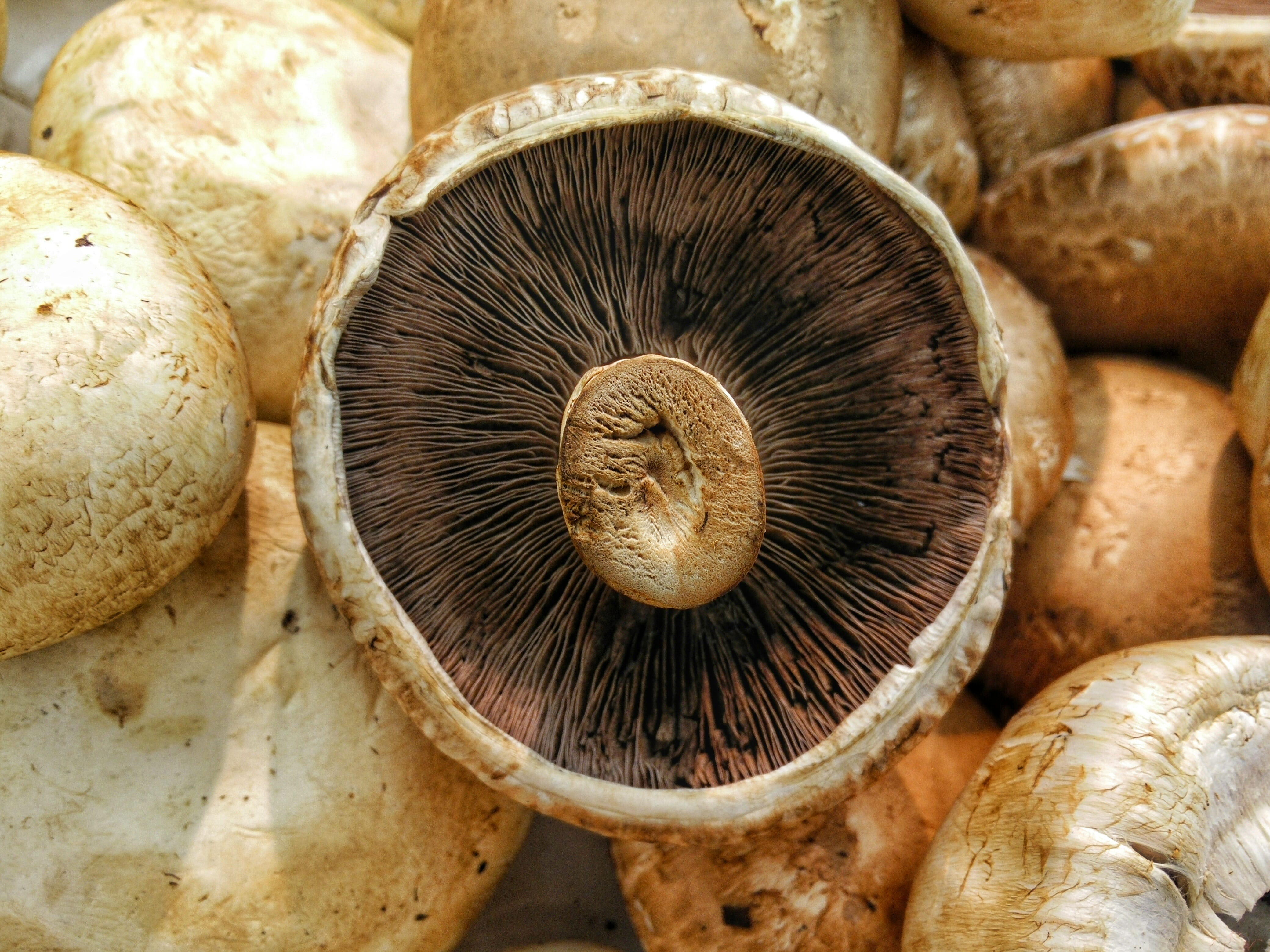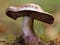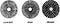# Chi-Squared Test for IndependencePhoto by Harshal S. Hirve on Unsplash

Pearson’s chi-squared test for independence is used to test whether there is an association between categorical variables by seeing if there is a statistical difference between the expected counts against the observed. The test uses the aggregated counts of the categorical variables that summarize the data into a table called a contingency table. Like other hypothesis testings, the chi-squared test proves by disproof by first assuming the expected counts are identical to observed(actual counts). If the probability of the observed being equal to expected is less than some set alpha, I reject the null in favor of the alternative; the expected counts are not equal to observed; therefore, the target variable depends on the discrete independent variable.

For this blog, I will use the UCI Mushrooms dataset that can be found here. (https://archive.ics.uci.edu/ml/datasets/mushroom). The dataset contains 22 discrete features of a mushroom species that explain if the species is either edible or poisonous(target variable). The dataset needs some preprocessing to translate feature codes to actual names, the data import and preprocessing can be done as such:

The data can be summarized using the pandas cross tab helper function to create a 2x2 contingency table.

Mushroom bruising involves nicking the top and bottom of the mushroom cap and observing any colour changes. As specimens that are not fresh don’t give reliable results, it is important to do this within the first 30 minutes of picking the mushroom. — https://mushly.com/discover/how-to-identify-mushrooms-through-bruising-and-bleeding#:~:text=Mushroom%20bruising%20involves%20nicking%20the,minutes%20of%20picking%20the%20mushroom.Blue Bruising: https://www.first-nature.com/fungi/images/cortinariales/cortinarius-purpurascens1.jpg

The objective is to test if mushrooms that are edible/not edible are dependent on the bruised condition of the mushroom. Firstly, state the null hypothesis, the bruised condition of the mushroom is independent of whether the mushroom is edible/poisonous, and the alternative, the bruised condition is not independent of the mushroom being edible. Secondly, state the alpha value of which will be used to determine the critical value. If the chi-squared statistic is greater than the critical value, the null hypothesis is rejected in favor of the alternative.

The observed counts are the aggregated counts of each variable that can be sliced using the Pandas helper function. The expected counts are the row-wise total multiplied by the column-wise total divided by the total number of observations. For example, the observed counts for bruised and edible(located on the top left of the contingency table) is 2752 and the expected counts are (3376*4208)/8124.

The chi-square statistic is the summation of the difference between observed and expected squared divided by the expected. For this example, the chi-square statistic is approximately 2037.50.

The critical value can be found here (https://www.itl.nist.gov/div898/handbook/eda/section3/eda3674.htm) by looking up the assigned alpha on the column and the degrees of freedom on the row. For an alpha of 0.05 and d.f. = 1, the critical value is 3.841.

Since the chi-squared statistic is greater than the critical value, I reject the null hypothesis that the expected counts are equal to the observed in favor of the alternative that the expected counts are not equal to the observed.

More simply, I can run a chi2 proportions test of independence using scipy.stats.chi2_contingency function. The function returns a tuple of 4 values, in the following order:
0. chi-squared statistic
1. p-value
2. degrees of freedom
3. expected counts.

To further analyze this dataset, I want to explore an additional independent variable, gill_spacing, with the same dependent variable, edible/inedible. A three-way contingency table is needed; however, I will hold one of the variables constant to create multi-partial tables to run multiple chi-squared tests when testing.https://midwestmycology.org/identify/

The idea is to take partial tables of this three-way contingency table by holding the gill_spacing feature constant. The result will be two partial tables; one for gill_spacing: Close and gill_spacing: Crowded.

Hello! My name is Albert Um.

Hello! My name is Albert Um.